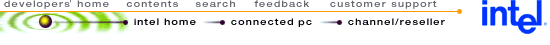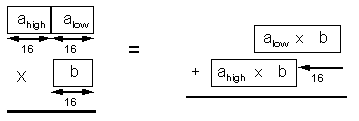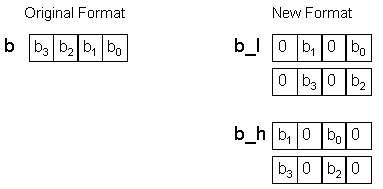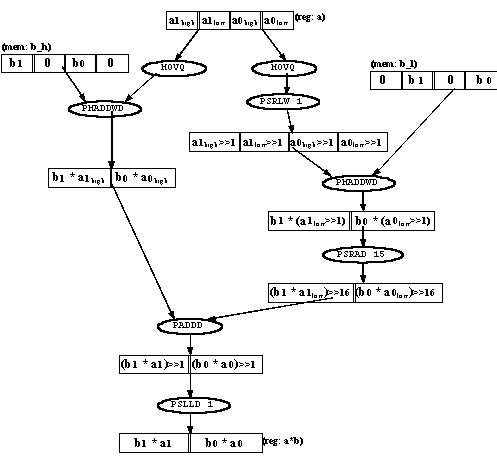## Using MMX™ Instructions to Perform 16-Bit x 31-Bit Multiplication

 Disclaimer Information in this document is provided in connection with Intel products. No license, express or implied, by estoppel or otherwise, to any intellectual property rights is granted by this document. Except as provided in Intel's Terms and Conditions of Sale for such products, Intel assumes no liability whatsoever, and Intel disclaims any express or implied warranty, relating to sale and/or use of Intel products including liability or warranties relating to fitness for a particular purpose, merchantability, or infringement of any patent, copyright or other intellectual property right. Intel products are not intended for use in medical, life saving, or life sustaining applications. Intel may make changes to specifications and product descriptions at any time, without notice. Copyright © Intel Corporation (1996). Third-party brands and names are the property of their respective owners.

 1.0. INTRODUCTION 2.1. Background 2.2. MMX Code Implementation 2.2.1. Data Format 2.2.2. Instruction Flow 3.1. Additional Optimizations 3.2. Comparison with Other Implementations

## 1.0. INTRODUCTION

The Intel Architecture (IA) media extensions include single-instruction, multi-data (SIMD) instructions. Because MMX technology uses SIMD instructions, four operations can be performed with one instruction. MMX technology does not include native support for the higher-precision multiply required by some applications. In many cases increasing the precision of one of the operands to 32 (or 31) bits is sufficient.

This document describes an implementation of a 16-bit by 31-bit multiplication operation using the MMX instructions. On some processors (such as the Pentium® processor), this implementation is significantly faster than using the IMUL instruction and is comparable in speed to the floating-point multiply instructions.

## 2.0. 16-BIT x 31-BIT MULTIPLICATION

This document presents an example of a routine which is optimized for use in a specific context: the case where one 16-bit matrix is multiplied with a large number of different 31-bit vectors. For different contexts, the code should be altered to achieve optimal performance. Additional assumptions were made about the data format, however, the code is easily altered to accommodate different formats (for example, changing the location of the radix point).

Assumptions which affect the multiplication routine code:

The same set of 16-bit multiplicands will be used for many multiplication operations. Therefore, their format in memory can be changed to improve performance. (The data is reformatted once and used over many multiplication operations.)

The 31-bit multiplicand is located in a register at the start of the computation and the result will be placed in a register at the end. The 16-bit multiplicand is in memory.

The 31-bit multiplicand is signed, with one sign bit, a 15-bit whole part, and a 15-bit fraction. The least significant bit is not used. The result is also 31 bits with the same format.

The 16-bit multiplicand is signed, with one sign bit, no whole part, and a 15-bit fraction.

### 2.1 Background

To understand the method used for 16-bit x 31-bit multiplication, it is necessary to understand the issues involved in performing this type of multiplication using MMX instructions. The following are the constraints:

MMX technology only has native support for 16x16-bit multiplications (with 32-bit results).

A 16x32-bit multiplication can be implemented using two 16x16-bit multiplications, a shift, and a 48-bit addition (see Figure 1).

Figure 1. Implementing a 16x32-bit Multiplication Using 16x16-bit MultiplicationsNote that the result of the 48-bit addition is truncated to 32 bits. It is possible to use a 32-bit addition by shifting the low partial result to the right instead of shifting the high partial result to the left. This introduces a small error, since truncation is performed before the addition instead of after it. However, the magnitude of this error is limited to the least significant bit of the result.

There is a problem in directly implementing this approach using the MMX technology multiply instructions. The number alow is unsigned and must be multiplied as such, but MMX technology only supports signed multiplication.

There are several possible solutions to this. The fastest solution is to logic-shift alow to the right by one bit before multiplication, and then to shift the multiplication result one bit to the left. This solves the signed-unsigned problem, because after the shift the most-significant bit of alow equals zero. In this case there is no difference between signed and unsigned multiplication. The disadvantage of this approach is that the least significant bit of alow is lost, so the multiplication is 16x31-bit instead of 16x32-bit. In many cases, this is acceptable.

Note that alow * b is shifted left by one bit, and then shifted right by 16 bits. Note that one shift to the right by 15 bits produces the same result.

### 2.2 MMX Code Implementation

This algorithm can be implemented in MMX technology with 2X parallelism (operating on two numbers at a time), since the operations are 32 bits wide (see Figure 1).

When multiplying 16-bit numbers in MMX code, there are two choices -- the PMADDWD instruction and the PMULL instructions. If a 32-bit result is required (as in this case), the PMADDWD instruction should be used. PMADDWD performs four multiplications and two additions. Since only two multiplications are required, half of each doubleword (one word) in one of the operands should be zero. The data format of b will be changed ahead of time so that extra operations are not needed during the multiplication (see Section 2.1).

### 2.2.1. Data Format

The 16-bit multiplicands (b) are assumed to be in a modified format. The new format is in Figure 2.

Figure 2. New Format for 16-bit MultiplicandsNote that the numbers are copied into two different locations. In the b_l location, the numbers are padded with zero so that they are in the low half of each doubleword. In the b_h location, the numbers are padded with zero so that they are in the high half of each doubleword where they are padded (in different ways) with zero.

In this new format, the 16-bit data takes four times the memory space compared to a scalar implementation (if the data was previously 32 bits then the new format will take up twice the previous space).

### 2.2.2. Instruction Flow

The instruction flow for the 16x31-bit multiplication appears in Figure 3.

Figure 3. 16x31-bit Multiplication Instruction FlowThe two MOVQ instructions are needed to preserve the value of a, which is in a register. Note that an additional shift operation is performed at the end, to adjust the result to the desired fixed-point format. This shift implies that the precision of the result is 31 bits, instead of 32.

Full 32-bit precision can be preserved in the result by moving the PSLLD 1 instruction to just after the PMADDWD a, b_h instruction, and changing the PSRAD 15 instruction to PSRAD 14. If 31-bit precision in the result is sufficient, leaving the PSLLD 1 instruction at the end might enable additional performance improvement (see Section 3.0).

## 3.0. PERFORMANCE

This code sequence performs two 16x31-bit multiplications in eight instructions. In many cases (for example, matrix-vector multiplication), the clock count for executing one sequence is less important than the throughput which can be achieved when many operations are scheduled in parallel. If the instructions for several 16x31-bit multiplies and other operations (loads, stores, adds, etc.) can be scheduled in parallel so as to achieve (on the Pentium processor) 100% pairing with no stalls, then every two multiplications will take four clocks (two clocks per 16x31-bit multiply).

There are good chances for achieving this optimal scheduling, since each 16x31-bit multiplication uses only two MMX registers. Therefore, instructions for three or four such operations can be intermixed without register conflicts, exposing many scheduling opportunities. Note that there are only two instructions in the code sequence which access memory, so memory-access instructions will not limit pairing. Due to these factors, performance of 2 to 2.5 clocks per 16x31-bit multiplication (on the Pentium processor) is achievable in many applications.

The PSLLD 1 instruction at the end of the code sequence can be avoided in many cases. This is possible in applications (such as vector and matrix multiplications, convolution operations, etc.) where many multiplication results are added together to produce a final result. In this case, all intermediate calculations may be performed on the unshifted numbers and the final shift performed only on the final result. This may reduce another 1/2 clock per multiplication operation - enabling a performance of two clocks or less per 16x31-bit multiplication.

### 3.2 Comparison with Other Implementations

In integer, scalar code, the IMUL instruction is an alternate method of performing multiplications. On the Pentium processor, it is about six times slower than the two clocks required for the MMX technology implementation.

Another alternative is using the floating-point instructions: either converting data to/from integer format on the fly using the FILD and FIST instructions, or converting all the code and data to floating-point. The floating-point multiply instruction, FMUL, has a latency of three clocks but is pipelined; other instructions can be scheduled in the meantime. Thus it is often possible to schedule instructions so that each FMUL instruction will take about 1 clock. This is twice as fast as the MMX technology implementation, but many factors may reduce this:

If many FILD and FIST instructions are used, performance will be much reduced.

Other operations (loads, adds, stores) tend to be faster on MMX technology than on floating-point operations, so the complete calculation may be the same or faster on MMX technology even though the 16x31-bit multiplication is slower than the floating-point multiplication.

The bottom line is that in many multiply-intensive operations, such as matrix-vector multiplication, this method enables the performance of the complete operation (on the Pentium processor) to be the same or better than floating-point code in cases where 16x31-bit precision multiplications are required.

## 4.0. CODE LISTING

Note that no attempt has been made to schedule the instructions for performance, since scheduling should be performed after intermixing several such code streams (using different registers) to expose more scheduling opportunities.

```TITLE   mx16x31
.486P
.model FLAT
.data
.const
.code
;****************************************************************
;void mx16x31 (
;    int   *a32,
;    int16 *bl16,
;    int16 *bh16 ) ;
; This function recieves a pointer to two signed 31-bit numbers,
; and to two signed 16-bit numbers (in a special format).
; It performs two 16x31-bit multiplies and writes the 31-bit
; result over the original 31-bit inputs.
; Data formats:
; a31 is in the format:
; (sign)(15-bit whole part).(15-bit fraction)(1 LSB is ignored).
; The 16-bit numbers are in the format:
; (sign).(15-bit fraction).
; In addition, the data is stored in the following way:
; bl16: b0,0,b1,0,b2,0,b3,0,....
; bh16: 0,b0,0,b1,0,b2,0,b3,....
;****************************************************************
mx16x31 PROC NEAR C USES ebx,
a32:PTR SDWORD, bl16:PTR SWORD, bh16:PTR SWORD
mov		eax,	a32
mov		ebx,	bl16
mov		ecx,	bh16	; Load a31 from memory

movq		mm6,	[eax]

; Here the code for the actual 16x31-bit multiplication starts.
; Note that the code is NOT scheduled for performance.
; This is because it should be scheduled together with
; other operations (two or three 16x31-bit multiplications should
; be scheduled, together with other operations).
BEGIN_MUL:
; Here a31 is in a register
movq		mm0,	mm6		; Copy a31
psrlw		mm0,	1		; Shift (for signed multiplication)
pmaddwd	mm0,	[ebx]			; Multiply b with low part of a31
movq		mm1,	mm6		; Copy a31
pmaddwd	mm1,	[ecx]			; Multiply b with high part of a31